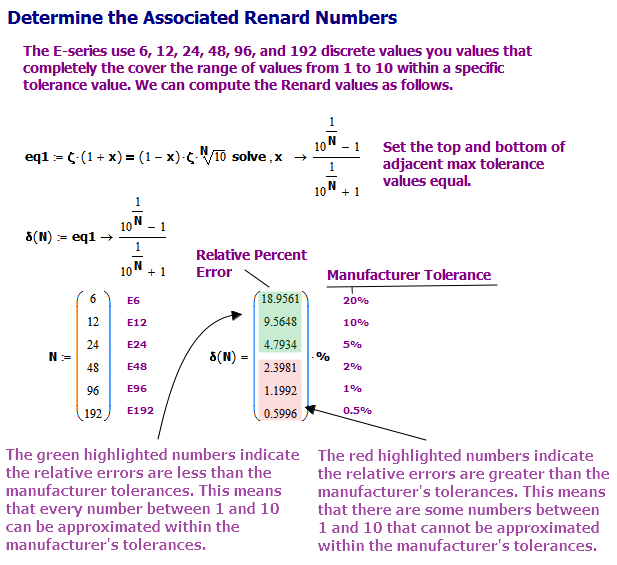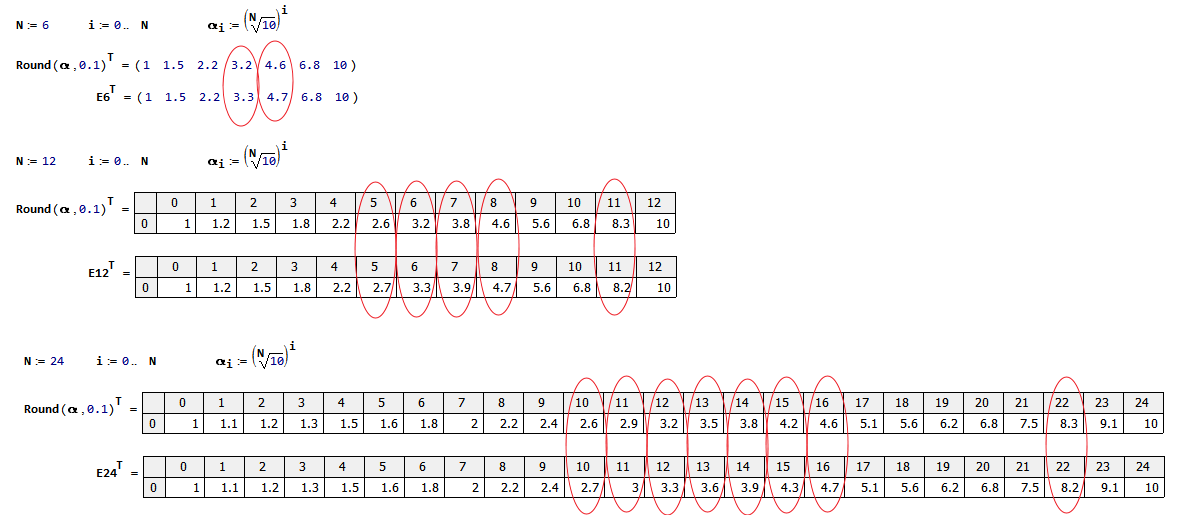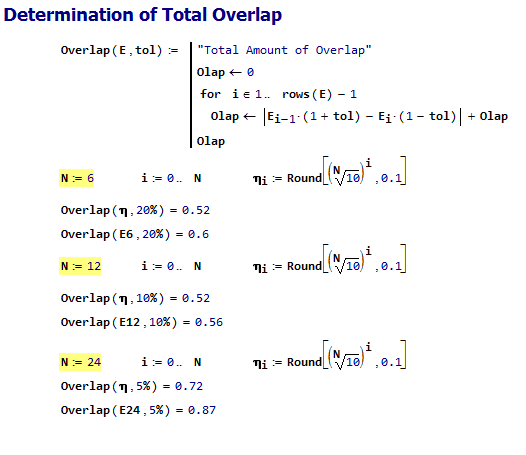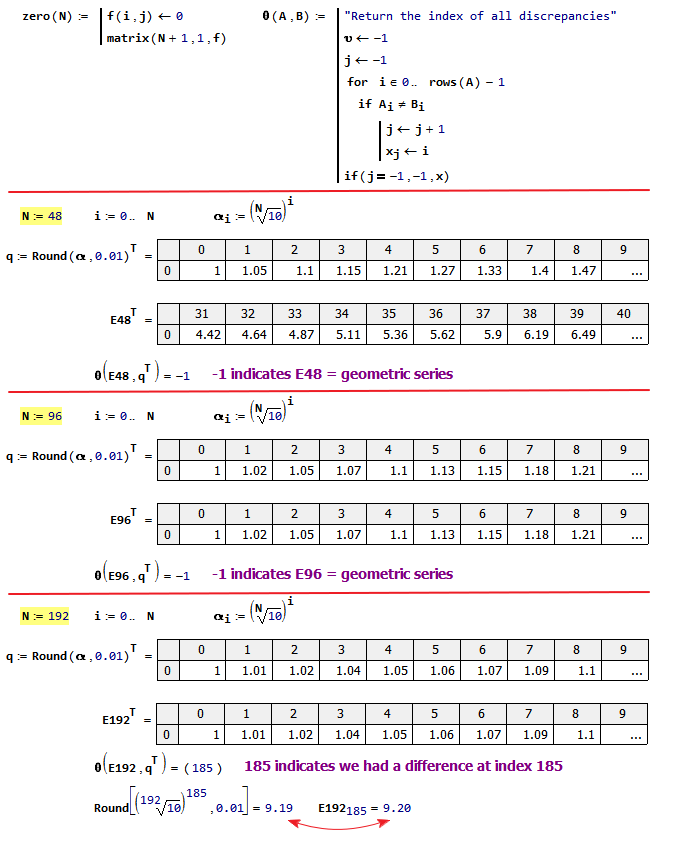# Standard Resistor Values

Quote of the Day

Never interrupt someone doing something you said couldn't be done.

— Amelia Earhart

## Introduction

I have been designing circuits with resistors since I was a kid working on science fair projects – I still remember building my first Radio Shack photocell project. While I have always thought of resistors as simple devices, I recently discovered that I have been laboring under a misconception about the standard resistor values.

Until last week, I believed that the values of the E-series standard resistor values were selected to ensure that if I needed a resistor within x% of a specific value, I simply needed to choose a resistor from the  x% tolerance set. For example, Figure 1 shows the E12 series (i.e. ±10%) values – notice that each tolerance range overlaps the adjacent ranges. This means that you can always find an E12 resistor value within 10% of your required value.

I was a bit surprised that I could not find an E48 value (±2% tolerance) within 2% of my design value. I was so surprised that I stopped what I was doing and learned how the E-series of standard resistor values were determined. It was an interesting side trip that I thought was worth discussing here.

When can you find an x% resistor within x% of a specific value? The answer is "it depends …"

• For 20% (E6), 10% (E12), and 5% (E24) resistors, you can always find a standard resistor value within 20%, 10%, or 5%, respectively, of the value you want.
• For the 2% (E48), 1% (E96), and 0.5% (E192) resistors, you will NOT always be able to find a standard resistor value within 2%, 1%, or 0.5%, respectively, of the value you want.

My objective here is to demonstrate the issue and provide a couple of ways of dealing with it. This is not a big deal because I can just specify a 1% or 0.5% resistor to get closer to value that I need. I was just surprised that the E series standard allowed these gaps. The tolerance on a resistor value simply means that the manufacturer guarantees that a resistor value is within the tolerance % of that specific value. For a given series, it does not mean that you can find a specific resistor value within the tolerance range of a standard resistance value.

## Background

### Definitions

Tolerance
In engineering, tolerance is the permissible limit or limits of variation in some parameter of a system or component (Source). Tolerance is often, but not always, expressed as the percentage of variation permitted from a specified value. All system parameters are subject to random variation, and the designer must deal with it.
Relative Percent Error (AKA Approximation Error)
The relative percent error (symbol δ) in the percentage discrepancy between an exact value and some approximation to it (Source). We normally compute the relative percent error with the equation $latex \delta =100\cdot \left| {\frac{{{{x}_{{approx}}}-x}}{x}} \right|&s=-1$, where x is the desired value and xapprox is the approximate value.
Preferred Number
Preferred numbers are standard guidelines for choosing exact product dimensions within a given set of constraints (Source).
Renard Numbers
Renard's system of preferred numbers, adopted in 1952 as international standard ISO 3, divides the interval from 1 to 10 into 5, 10, 20, or 40 steps. The factor between two consecutive numbers in a Renard series is approximately constant (before rounding), namely the 5th, 10th, 20th, or 40th root of 10 (approximately 1.58, 1.26, 1.12, and 1.06, respectively), which leads to a geometric sequence. This way, the maximum relative error is minimized if an arbitrary number is replaced by the nearest Renard number multiplied by the appropriate power of 10 (Source).
E-Series
In electronics, IEC 60063 defines a preferred number series for resistors, capacitors, inductors, and Zener diode voltages that subdivides the interval from 1 to 10 into 6, 12, 24, 48, 96, and 192 steps (similar in approach to the Renard numbers). These subdivisions ensure that when some arbitrary value is replaced with the nearest preferred number, the maximum relative error will be on the order of 20%, 10%, 5%, 2%, 1%, 0.5% (Source).

The yellow highlighted text is the important thing here – the maximum relative error only roughly corresponds to the tolerance – you are not guaranteed to have a preferred number within the tolerance range of the resistor series.

I should also mention that the actual E-series values do not always follow the geometric relation $latex {{x}_{i}}={{\left( {\sqrt[N]{{10}}} \right)}^{i}}$, where N is the series number and i=0 … N-1. The E6, E12, and E24 series have had some their values moved around a bit (Appendix A). The E48 and E96 series exactly match the geometric series. The E192 series has only one discrepancy –  9.20 instead of the geometric series value of 9.19 (Appendix B).

## Analysis

### Absolute Error Versus Tolerance

Figure 2 shows the maximum relative error you will see for a given manufacturer's tolerance specification. Observe that the E48, E96, and E192 series have tolerances less than the maximum relative error.Figure 2: Difference Between Relative % Errors and Manufacturing Tolerances.

### Graphical View

#### E6 Example Showing Full Coverage From One to Ten

Figure 3 shows a set of bars that illustrate the range of values covered each resistor value in the E6 series. Note that each resistor range overlaps with the adjacent resistor ranges. This means that any value in the range 1 to 10 can be covered by a E6 value within 20%.

#### E48 Example Showing Gaps For Some Numbers Between One and Ten

Figure 4 shows graph similar to Figure 3, but for the E48 series (±2%). It is difficult to see at this scale, but there is not a standard value for every value from one to ten within 2%.

We can magnify the scale on Figure 4 and show an example of the gaps that exist. For a concrete example, consider the number 8.455. It is 2.5% away from 8.2 and 2.4 % away from 8.66, the two nearest E48 values.

Again, this is not a big deal because we can work around this issue. However, I was just surprised to learn this after all these years.

### Workarounds

The easiest workaround is just to use a resistance series with a finer resolution. In my case here, I wanted to use the E96 series. I could also have used the E192 series, which would have solved the problem.  I should mention that some folks use multiple resistors to "tweak" a value. Here are some example circuits (Figure 6) from W. Stephen Woodward. I have put out a blog post on how to select the correct standard resistor values to bring you with 0.1% of any resistor value between 10 Ω and 1 MΩ.

## Conclusion

The Renard numbers and their E-series variants are used for all sorts of components, including capacitors, inductors, and Zener diodes. This exercise was useful because it showed me that there is stuff to learn even in something that I have been using for years.

## Appendix A: E6, E12, E24 Series Geometric Variances

Figure 7 shows the differences (marked with red ovals) between the E6, E12, and E24 values and the associated geometric series.Figure 7: Red Circles Mark Differences Between E-Series and Geometric Series.

I do not know why the E6, E12, E24 values were not set equal to the geometric series values. I speculate that modifying the geometric values slightly improved some characteristic important to manufacturing. For example, the total overlap between adjacent values is larger for the E series values than for the geometric series values. This likely reduces the relative error and may improve yields for difficult to control parameters like Zener diode voltages that also use the E series.Figure 8: Total Overlap is Larger with E Series Values Than Geometric Series Values.

## Appendix B: E48, E96, E192 Series Geometric Variances

In Figure 9, I show that among the series values for E48, E96, E192, there is only one discrepancy between the standard values and the associated geometric series (9.19 vs 9.20).Figure 9: One Discrepancy Between E48, E96, E192 and the Associated Geometric Series.

Save

This entry was posted in Electronics. Bookmark the permalink.

### 8 Responses to Standard Resistor Values

1.Gene Nelson, Ph.D. says:

Thank you for an informative blog post regarding a (supposedly mundane) topic.

•mathscinotes says:

I often worry that some of my posts are too mundane, but I believe in documenting what I learn in the hope that it may be useful to other folks. Some of my most popular posts (e.g. computing required spare part inventories) were almost not posted because I thought " who would care?" Now I just post everything.

mathscinotes

2.Gert Willmann says:

A reasonable explanation for the discrepancy of the E192 value at index 185, which is standardized as 920 instead of the mathematically correct value 919, might be as follows:

Both the next-lower and the next-higher values, that is, the E96 values 909 and 931, are heavily rounded up from 908.5176 and 930.5720, respectively, whereas the ‘correct’ value 919 would have been heavily rounded down from 919.4787. This is the only position within the E192 series where such an unfavorable round-off sequence occurs. Therefore, those who actually standardized the E192 series might have decided to sacrifice the strictly logarithmic sequence of (rounded) values at this particular position in order to obtain a much smoother overall behavior of the E192 series.

I haven't found an official confirmation of this, though.

•mathscinotes says:

Hi Gert,

That does sound like the kind of practical reasoning that would go into making a change like this. Thanks for the comment.

mark

•Gert Willmann says:

Hi Mark,

I'd like to submit a lengthy reply to one of your other blog posts dealing with resistor combinations. As this reply would contain a few lists, simple tables, and also a small ASCII graphic, it would be necessary to include the respective HTML tags.

In particular, I would need the ‘ul’ and ‘ol’ tags (for unordered/ordered lists), ‘code’ (for small inline code snippets), ‘pre’ (for ASCII tables and for the ASCII graphic), and ‘sup’ (for exponents); a few special symbols such as ‘≤’ (less than or equal) would be useful, too. And what about links to other sites? I've left out the angle brackets here to avoid unwanted side-effects in case that HTML is already supported.

I have no problem to view the source code of any existing page and to extract whatever I need for my purposes. I just don't know whether it's possible to include HTML tags in a reply, and if so, how to do this.

Could you clarify this and perhaps give me a few hints, please?